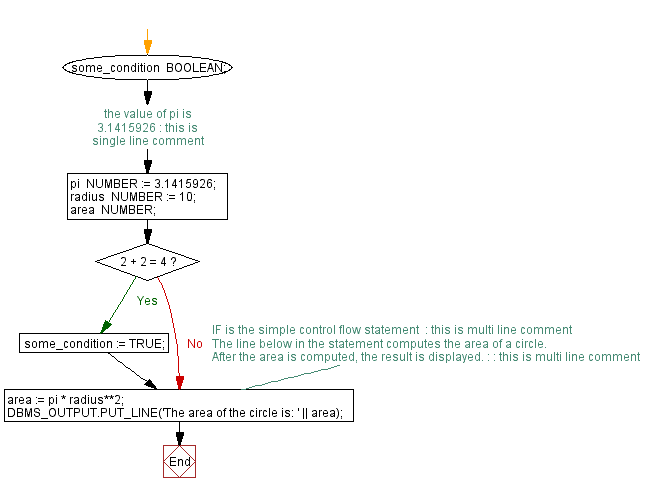﻿ PL/SQL Fundamentals Exercises: PL/SQL block to show Single and Multiline Comments - w3resource# PL/SQL Fundamentals Exercises: PL/SQL block to show Single and Multiline Comments

## PL/SQL Fundamentals: Exercise-6 with Solution

Write a PL/SQL block to show single and multiline comments.

PL/SQL Code:

``````DECLARE
some_condition  BOOLEAN;
pi              NUMBER := 3.1415926; -- the value of pi is 3.1415926 : this is single line comment
radius          NUMBER := 10;
area            NUMBER;
BEGIN

IF 2 + 2 = 4 THEN
some_condition := TRUE;
/* IF is the simple control flow statement  : this is multi line comment*/
END IF;

/* The line below in the statement computes the area of a circle.
After the area is computed, the result is displayed. : : this is multi line comment*/

area := pi * radius**2;
DBMS_OUTPUT.PUT_LINE('The area of the circle is: ' || area);
END;
/
```
```

Sample Output:

```The area of the circle is: 314.15926

Statement processed.

0.00 seconds
```

At the time of testing or debugging a program, you can disable a line of code by making it a comment. For example:

``````
-- DELETE FROM EMPLOYEES WHERE FIRST_NAME='Lex';
```
```

Flowchart:Improve this sample solution and post your code through Disqus

What is the difficulty level of this exercise?

﻿